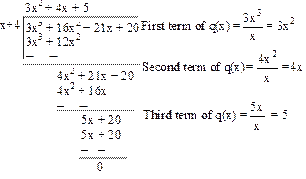# Mathematics

### Chapter : Polynomials

#### Working Rule to divide a Polynomial by Another Polynomial

Working Rule to divide a Polynomial by Another Polynomial

Step 1: First arrange the term of dividend and the divisor in the decreasing order of their degrees.
Step 2 : To obtain the first term of quotient divide the highest degree term of the dividend by the highest degree term of the divisor.
Step 3 :  To obtain the second term of the quotient, divide the highest degree term of the new dividend obtained as remainder by the highest degree term of the divisor.
Step 4 : Continue this process till the degree of remainder is less than the degree of divisor.

Division Algorithm for Polynomial
If p(x) and g(x) are any two polynomials with g(x) ≠ 0, then we can find polynomials q(x) and r(x) such that
p(x) = q(x)×g(x)+r(x)
where r(x) = 0 or degree of r(x) < degree of g(x).

The result is called Division Algorithm for polynomials.

DIVIDEND = QUOTIENT × DIVISOR + REMAINDER

Example: Divide 3x3 + 16x2 + 21x + 20 by (x + 4).

Solution:Quotient = 3x2 + 4x + 5 and Remainder = 0

Example: Apply the division algorithm to find the quotient and remainder on dividing p(x) by g(x) as given : p(x) = x3 – 3x2 + 5x – 3, g(x) = x2 – 2

Solution: We have,
p(x) = x3 – 3x2 + 5x – 3 and g(x) = x2 – 2We stop here since degree of (7x – 9) < degree of (x2 – 2). So, quotient = x – 3, remainder = 7x – 9, Therefore,
Quotient × Divisor + Remainder
= (x – 3) (x2 – 2) + (7x – 9)
= x3 – 2x – 3x2 + 6 + 7x – 9
= x3 – 3x2 + 5x – 3 = Dividend
Therefore, the division algorithm is verified.